MathExamle.com#### Welcome to the site MathExample.com

This educational resource is intended for you to acquire skills in practical mathematics, as well as for the development of mathematical abilities in you and your children.

" If people do not believe that mathematics is simple, it is only because they do not realize how complicated life is "

~John von Neumann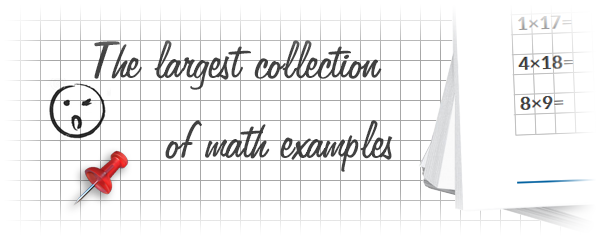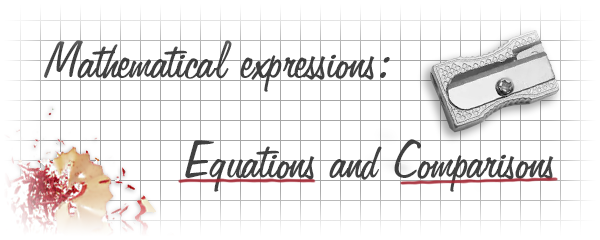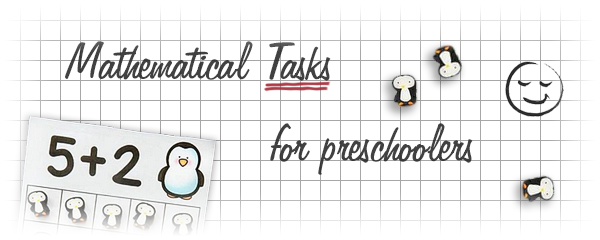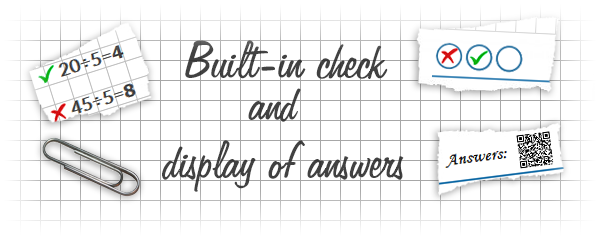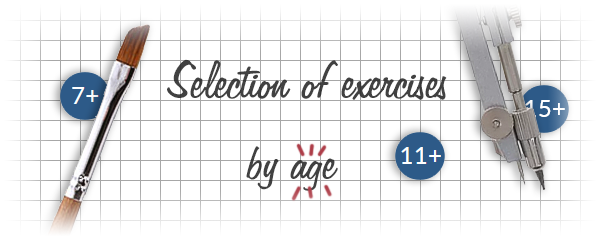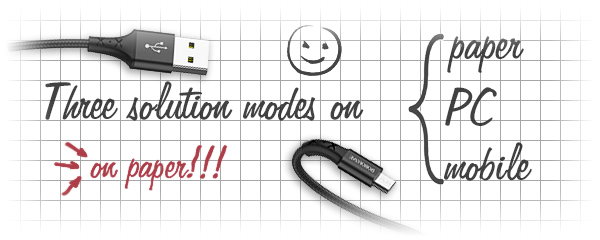Website Goals:

• increased knowledge in mathematics
• gaining practical experience in solving mathematical tasks
• increasing the speed and quality of logical and mathematical thinking
• development of memory, mindfulness and concentration

Using this resource you can:

• gain basic knowledge of addition, subtraction, multiplication and division
• learn the multiplication table with practical exercises
• learn operations with integer, decimal and fractional numbers
• master the methods of solving equations
• get hands-on spelling

The site provides the following features:

• creation of tasks of any complexity
• printing worksheets of any size
• solving and fixing results online
• built-in check and display of answers by link or QR code
• two modes on a computer and on a mobile device

The ability to think rationally needs to be developed and consolidated, it is easier to do with us!

6+

Create worksheets of  mathematical examples with addition and subtraction. The numbers of a example are integer. The answers are always positive and integer. Total 5 variants and 5 levels of complexity.

Creates examples with addition and subtraction operations of integers, the answers are always positive

5 Variants 5 Levels
 8-6+8= 5+4-3= 3+7-4= 10-2-5= 10-3-4= 10-1-2=
Variant: 4 Level: Easy
 Sheet
10+

Creates worksheets with English infinitives where some words are missing. Missing verbs must be completed. Only 3 options and 3 difficulty levels.

Creates worksheets with English infinitives where some words are missing, missing verbs must be completed

3 Variants 3 Levels
 rid rid [rid] outleap outleaped [outleap]
Variant: 1 Level: Easy
 vocabulary Sheet
6+

 4+4= 4+8= 4+1=
Attempts: 2 Level: Easy
10+

Create worksheets of mathematical examples for addition and subtraction by a column method. The answers and numbers of a example are integer and positive. Total 3 variants and 3 levels of complexity.

Creates examples for addition or subtraction of integers by a column method

3 Variants 3 Levels
 120000+793277= 561815+138185= 883665+116335= 242327+357673=
Variant: 1 Level: Hard
 Sheet
14+

Create worksheets of quadratic equations. The numbers of a equation are integers. The variables can be negative. Total 3 variants and 3 levels of complexity.

3 Variants 3 Levels
 -6x2-48x=0 -5x2+10x=0 2x2-6x=0 4x2+24x=0 3x2-6x=0 x2+5x=0
Variant: 2 Level: Easy
 Sheet

## Visitors MathExample.com

 If you have any questions or requests to improve this site, please contact us. If errors occur on the site, please also write to our developers.